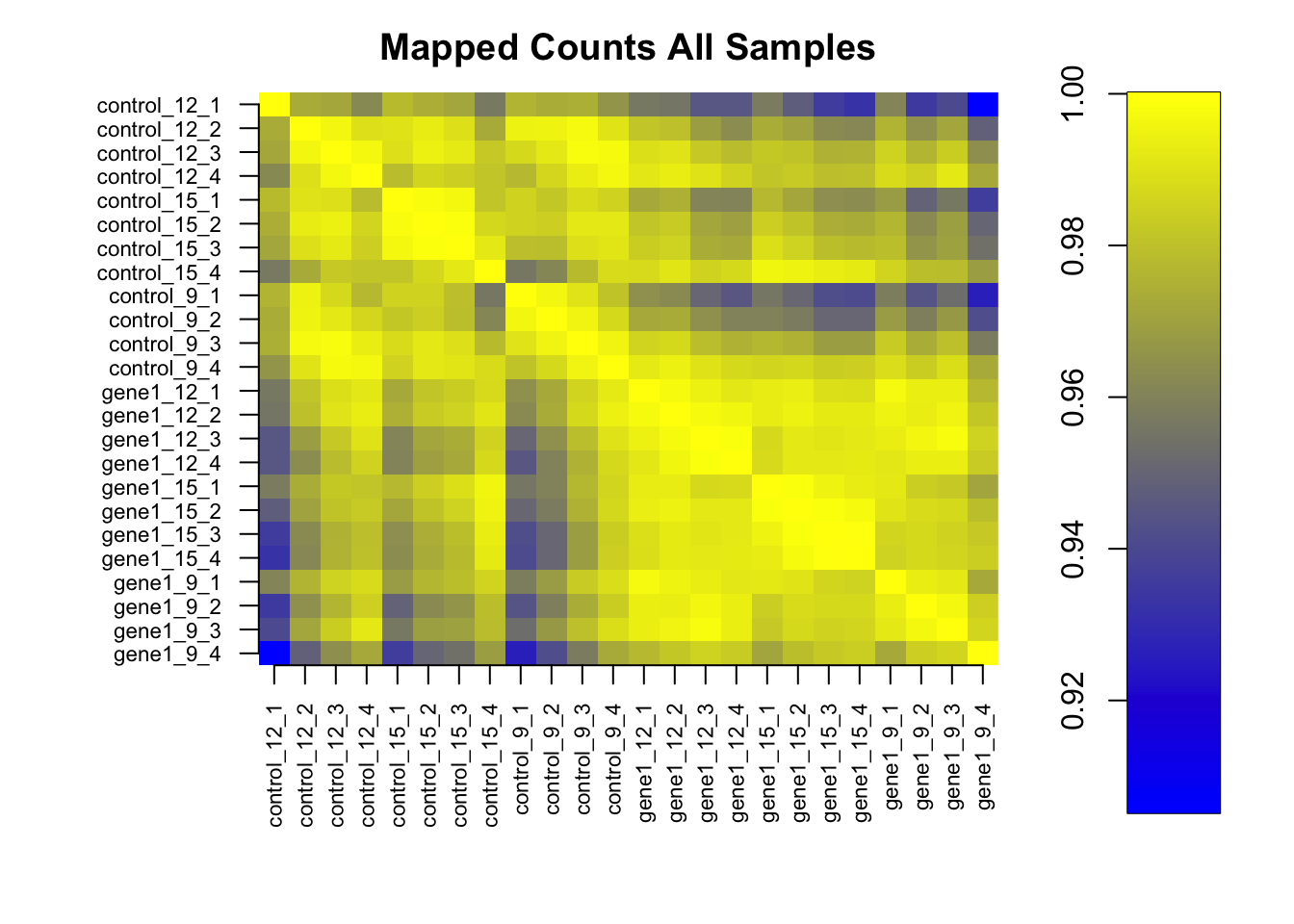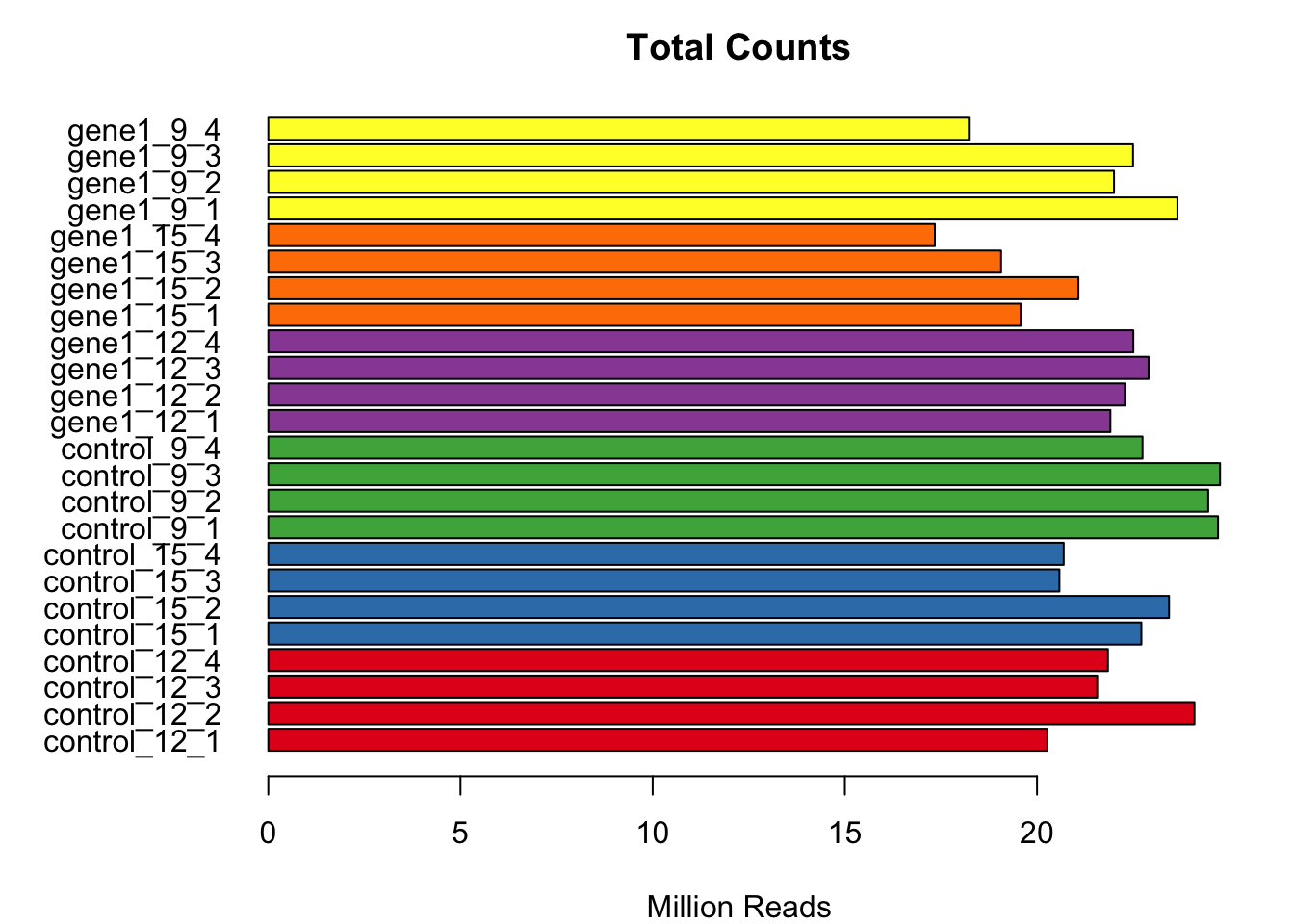## Overview

This is a script that will do differential gene expression (DGE) analysis for RNA-seq experiments using the bioconductor package edgeR.

## Project Details

This project is an RNA-seq experiment being done to look at the differential gene expression levels of an RNAi knockdown of the histone modifier ‘gene1’, compared to an RNAi control, ‘control’. This was done at three time points, day 9, 12 and 15 after RNAi exposure. As stated above, DGE analysis was done using the bioconductor package edgeR.

The first step is to load in the necessary packages that are going to be used in this experiment.

``````library(RColorBrewer)
library(genefilter)
library(edgeR)
library(ggplot2)
library(knitr)``````

## Load in Files And Combine Samples Used on Multiple Lanes

Next, I load in the counts table and combine the samples that are run on multiple lanes. This is done so that a sample has one column of counts for all genes.

``````setwd("~/Desktop/RNA.seq.example/")
### Read in the counts table

### Remove the lane from the column name so it just shows each sample name (gene1_12_1_6 -> gene1_12_1)
colnames(counts) <- sub("_[0-9]\$","",colnames(counts))``````
``````### Sum the counts from the aliquots (all lanes combined into one row)
scounts <- t(apply(counts, 1, function(x) {
tapply(x, factor(colnames(counts)), sum)
}))

### Test a couple of columns to check and make sure our counts table looks good``````
gene1_12_1 gene1_12_1.1 gene1_12_1.2 gene1_12_2 gene1_12_2.1
SMED30030523 19 21 20 11 19
SMED30018010 4 3 2 2 1
SMED30032462 2 4 0 1 0
SMED30024982 0 0 1 0 0
SMED30017564 92 116 102 132 142
SMED30034967 932 934 976 1019 983

## Project Summary Table

Following, I create a summary table that I can send back to my researcher that outlines what each sample is and the experimental factors involved.

``````### Creating a Samples Data frame
### Sample Column
Sample <- colnames(scounts)

### RNAi column
RNAi <- c(rep("control", 12), rep("gene1", 12))

### Replicate Column
Replicate <- rep(1:4,6)

### Day Column
Day <- c(rep(12,4), rep(15, 4), rep(9, 4), rep(12, 4), rep(15, 4), rep(9, 4))

### Create a name that is more structured
Alias <- paste(RNAi, Day, Replicate, sep="_")

### Creat Summary Table
targets <- data.frame(Sample, RNAi, Day, Replicate, Alias)

### Check to make sure it works
Sample RNAi Day Replicate Alias
control_12_1 control 12 1 control_12_1
control_12_2 control 12 2 control_12_2
control_12_3 control 12 3 control_12_3
control_12_4 control 12 4 control_12_4
control_15_1 control 15 1 control_15_1
control_15_2 control 15 2 control_15_2
``````### Write this file out for researcher
write.table(targets, "Samples.txt", quote=FALSE, row.names=FALSE, col.names=T, sep="\t")

### Change the sample names in our counts table to our more structured name (RNAi_Tisssue_Day_Replicate)
colnames(scounts) <- Alias``````

## Filtering Steps

The next step is to filter out unmapped and ribosomal reads, as well as genes with low read counts.

``````### Filter out unmapped reads designated "*"
mcounts <- scounts[-match("*", rownames(scounts)),]

### Get rid of main ribo transcript
mcounts <- mcounts[-match("SMED30032887", rownames(mcounts)),]

### Get rid of other ribo28s and 16s transcripts
mcounts <- mcounts[-which(rownames(mcounts) == "SMED30032663"),]
mcounts <- mcounts[-which(rownames(mcounts) == "SMED30027845"),]

### Filter out genes with low read counts
countData <- mcounts[rowSums(cpm(mcounts)) > 4,]``````

## Plot Stats About RNA-seq Library

Next, to ensure quality it is important to plot some statistics about the library. Specifically, I plotted the correlation between samples and a bar plot of the amount of mapped and total reads.

``````### Preload a predifined correlation plot function
source("~/Desktop/RNA.seq.example/myImagePlot.R")
myImagePlot(cor(cpm(countData)),
title= "Mapped Counts All Samples")````````````### Create a vector of total counts in millions for each sample to be plotted
totalCounts <- colSums(scounts[-match(c("SMED30032887",
"SMED30032663",
"SMED30027845"),
rownames(scounts)),])/10^6

###Create a vector of mapped counts to be plotted in Millions of Reads
mappedCounts <- colSums(mcounts)/10^6

### Create a vector of colors for each sample type using RColorBrewer
allSample.cols <- rep(brewer.pal(6, "Set1"), each=4)

### Change the margins so the sample names will fit
mymar <- par()\$mar
mymar <- 7
mymar <- 4
par(mar = mymar)

###Use the barplot function
barplot(totalCounts,
col = allSample.cols,``````barplot(mappedCounts,